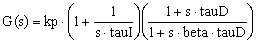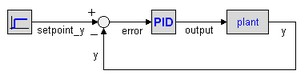﻿ 20-sim webhelp > Library > Signal > Control > PID Control > Discrete > pid

pid

Library

Signal\Control\PID Control\Discrete

Use

Domains: Continuous, Discrete Size: 1-D. Kind: Block Diagrams.

Description

This is a PID controller in series form. The transfer function is:with the input of the controller equal to the error and the output of the controller used as the plant input:Interface

Inputs

Description

error

Input signal: plant-output minus set point.

Outputs

output

Output signal: input for the plant.

Parameters

kp

tauD

tauI

beta

Proportional gain.

Derivative gain (tauD <> 0).

Integral gain (tauD <> 0).

Tameness constant (0 < beta << 1).

Initial Values

pdstate(0)

pdout(0) = pdstate(0) - kp*error(0)*/beta

pistate(0)

output(0) = pistate(0) - pdout(0)